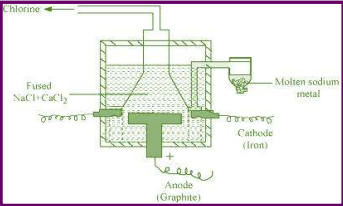Question 10.16:

Starting with sodium chloride how would you proceed to prepare

(i) sodium metal

(ii) sodium hydroxide

(iii) sodium peroxide

(iv) sodium carbonate?

(i) Sodium can be extracted from sodium chloride by Downs process.
This process involves the electrolysis of fused NaCl (40%) and CaCl2 (60 %) at a temperature of 1123 K in Downs cell.Steel is the cathode and a block of graphite acts as the anode. Metallic Na and Ca are formed at cathode. Molten sodium is taken out of the cell and collected over kerosene.
(ii) Sodium hydroxide can be prepared by the electrolysis of sodium chloride. This is called Castner–Kellner process. In this process, the brine solution is electrolysed using a carbon anode and a mercury cathode.
The sodium metal, which is discharged at cathode, combines with mercury to form an amalgam.
(iii) Sodium peroxide
First, NaCl is electrolysed to result in the formation of Na metal (Downs process). This sodium metal is then heated on aluminium trays in air (free of CO2) to form its peroxide.
(iv) Sodium carbonate is prepared by Solvay process. Sodium hydrogen carbonate is precipitated in a reaction of sodium chloride and ammonium hydrogen carbonate.
$2{\mathrm{NH}}_{3}+{\mathrm{H}}_{2}\mathrm{O}+{\mathrm{CO}}_{2}\to {\left({\mathrm{NH}}_{4}\right)}_{2}{\mathrm{CO}}_{3}\phantom{\rule{0ex}{0ex}}{\left({\mathrm{NH}}_{4}\right)}_{2}{\mathrm{CO}}_{3}+{\mathrm{H}}_{2}\mathrm{O}+{\mathrm{CO}}_{2}\to 2{\mathrm{NH}}_{4}{\mathrm{HCO}}_{3}\phantom{\rule{0ex}{0ex}}{\mathrm{NH}}_{4}{\mathrm{HCO}}_{3}+\mathrm{NaCl}\to {\mathrm{NH}}_{4}\mathrm{Cl}+{\mathrm{NaHCO}}_{3}$
These sodium hydrogen carbonate crystals are heated to give sodium carbonate.
$2{\mathrm{NaHCO}}_{3}\to {\mathrm{Na}}_{2}{\mathrm{CO}}_{3}+{\mathrm{CO}}_{2}+{\mathrm{H}}_{2}\mathrm{O}$